-=+=- -=+=- -=+=- -=+=- -=+=- -=+=- -=+=- -=+=- -=+=- -=+=- -=+=- -=+=- -=+=- -=+=- -=+=- -=+=- -=+=- -=+=- -=+=- -=+=- -=+=- -=+=- -=+=- -=+=- -=+=- -=+=- -=+=- -=+=- -=+=- -=+=- (c) WidthPadding Industries 1987 0|557|0 -=+=- -=+=- -=+=- -=+=- -=+=- -=+=- -=+=- -=+=- -=+=- -=+=- -=+=- -=+=- -=+=- -=+=- -=+=- -=+=- -=+=- -=+=- -=+=- -=+=- -=+=- -=+=- -=+=- -=+=- -=+=- -=+=- -=+=- -=+=- -=+=- -=+=-

Pakz# Blitz Basic Sprite Editor

24th August 2018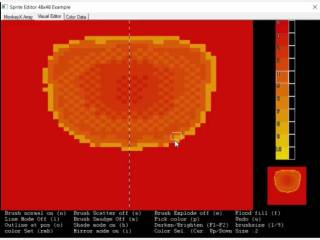Sprite Editor

Pakz# Fire Power

16th March 2018Fire Power

Pakz# Player vs Zombies or Armed Soldiers

14th October 2017Fight in dungeons against monsters/soldiers

Pakz# A Civilization Clone v0.75

21st June 2017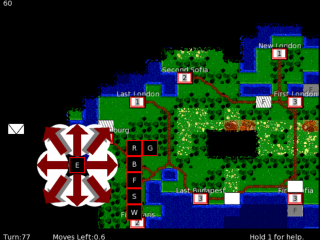Browser based turn based strategy game

Pakz# Roguelike Explorer

12th April 2017Simple Html5 exploration game with Touch support

Pakz# Between Space

18th December 2016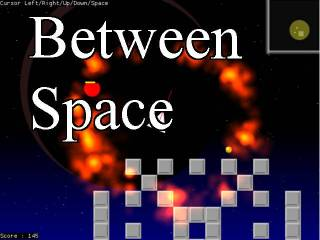2d topdown space shooter with pickups and drones

Pakz# Mining Map AI Demo

13th July 2016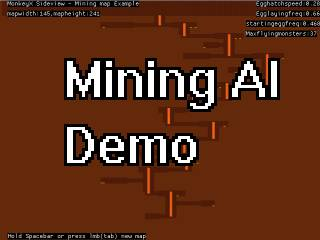A wip of a game with flying monsters in a mine.

Pakz# Grey Lined Backgrounds

11th July 2016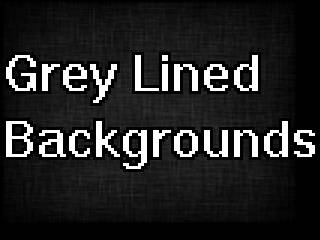Grey Texture Generator

Pakz# Map Generator variation

16th June 2016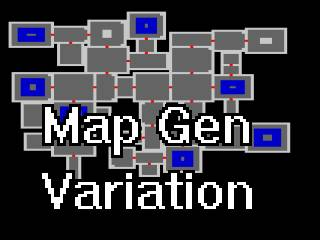Rooms and doors and things in rooms

Pakz# Grey backgrounds

22nd May 2016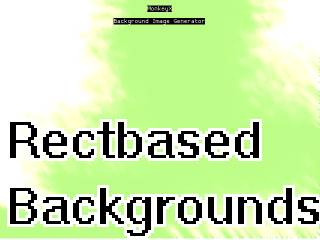Several graphical filters in action

Pakz# Mojo2 html5 animation

11th May 2016A nice looking background animation effect

Pakz# Blitzplus Pixeling Tool Project

24th April 2016

Create pixelart, various features, wip

More - Older Posts